Courses

# Blood Relationship and Coded Relationship CLAT Notes | EduRev

## Logical Reasoning for CLAT

Created by: Gyanm Institute

## Class 10 : Blood Relationship and Coded Relationship CLAT Notes | EduRev

The document Blood Relationship and Coded Relationship CLAT Notes | EduRev is a part of the Class 10 Course Logical Reasoning for CLAT.
All you need of Class 10 at this link: Class 10

BLOOD RELATIONSHIP AND CODED RELATIONSHIP
Questions in Blood Relations are inevitably asked in examination. The questions are related to our ‘relationships’ in the family. The examiner wants to test your ability to see through the cobweb. It is a test of intelligence and logic. The student is expected to find out ‘relationship’ between two person based on the given ‘input’.
Before we proceed further, let us understand clearly that all relationships are extension of three basic relationships.
1. First Basic Relationship is of Parent & Child
2. Second Basic Relationship is of Husband & Wife
3. Third Basic Relationship is of
Brother -----------Brother
or   Brother-----------Sister
or   Sister----------Sister
4. The gender of the persons whose relationship is to be found is also of utmost importance.
To solve the questions based on Blood relationships, we can represent them diagrammatically as follows

DIAGRAMMATIC REPRESENTATION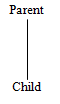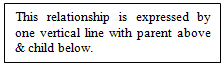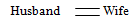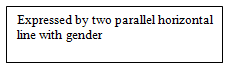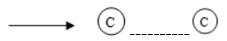This is expressed by one horizontal broken line with gender below it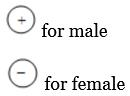We follow this as a standardized system so that there is no room for confusion. Based on above information, we can describe any relationship diagrammatically.
Generally two types of questions are asked in the examinations:
Type 1: Jumbled Relationships: These are based on direct relationships and the student is expected to see through and find out the exact relationship between the two persons.

Example 1. ‘My father’s only son’;
Solution. It means either the person himself or if the lady is talking to somebody, then she is talking about her brother.

Example 2. When the examiner says, “My son’s mother”,
Solution. It means the person is talking about his wife.

Example 3. A person state that I have no brother or sister and the person in this photograph is son of my father.
Solution.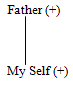So person is talking about himself & photograph is of his ‘own’.

Example 4. Pointing to a photograph, a person says that this is photograph of only daughter in law of his son’s mother. Whom is he talking about?
Solution. If we follow the statement and draw the diagram as follow:-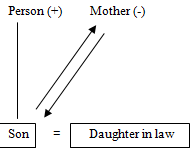(Person’s son’s mother is Person’s wife)
Mother’s only ‘Daughter in law’ means that she has only one son and ‘Daughter in law’ is wife of his own son. The person is talking about. So she is ‘Daughter in law’ of the person as well.

Example 5. Pointing to a photograph, a man says, that this is photograph of paternal grandfather of husband of his daughter in law. Whom is he talking about?
Solution. Read the question carefully and draw the diagram following the leads given above:
Grandfather of (+)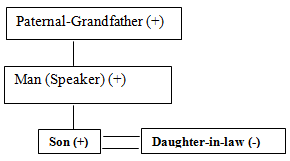(i) Man’s daughter in law is his Son’s wife.
(ii) Her husband is the son of that man (speaker)
(iii) Son’s grandfather is speaker’s father
(iv) Photograph is that of ‘father’ of man.

Example 6. A lady states that this is photograph of only daughter of ‘father- in – law’ of her husband. Whom she is talking about?
Solution. If we draw the diagram using above information, it follows as under:
(ii) We identify her husband.
(iii) We identify her husband’s father in law.
(iv) We identify that the daughter of husband’s father in law is the lady herself.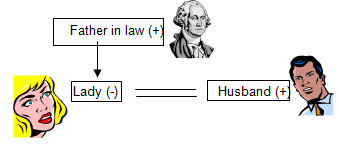Since father in law has only one daughter, the lady is wife of the husband she is talking about. It means that it is her own photograph.
Based on information given to us, it is possible to solve questions based on Blood Relationships easily in two ways:
(i) Pictorial ways
(ii) Analytical Method
The student should start with Pictorial method and after they have clearly understood the pattern, should follow Analytical method to solve the question quickly or easily.
The following chart will help you to solve questions in blood relationship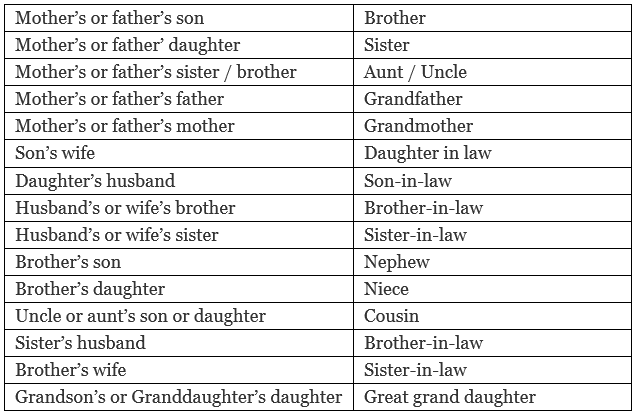Some Important Tips
(i) First of all choose the two persons, between whom relationship is to be established.
(ii) Next, pin-point the intermediate relationship i.e., such relationship through which long drawn relationship can be established between the required persons.
(iii) Finally, conclude the relationship directly between the two persons as per the requirement of the question.
Type 2: Coded Relationship: The relationship between the two persons is given in the form of a ‘symbol’. It may be given that A + B means that A is father of B.
It tells us that the symbol ‘+’ denotes the relation of ‘father’. But in this statement, it is given that it is A who is father of B and not vice versa. It does not mean that B is father of A and nor does it mean that B is son of A; for we do not know the gender of B. B may be either the son or daughter of A. Similarly, it may be given to us that A + B means that B is father of A.
Again the symbol ‘+’ stands for ‘father’. But here, it is B, who is father of A and not vice versa.
Thus the ‘Coded Relationship’ questions can be categorized into two types:
(i) Relation of the person on the left with the person on his right.
(ii) Relation of the person on the right with the person on his left.

TYPE I (Relation of the person on the left with the person on the right)
A + B means A is father of B.
A – B means A is mother of B.
In both these cases, we are given the relationship of A, who is on the left with the person B who is on his right. We start from right and move towards left. As such we call it ‘Relation – Back’.

Example: If it is given that:
(i) ‘A+B’ means ‘A is the father of B’
(ii) ‘A – B’ means ‘A is the mother of B”.
(iii) ‘A x B’ means ‘A is the brother of B’
(iv) ‘A ÷ B’ means ‘A is the sister of B’.
What is the relationship of P with R in the equation: ‘P + Q x R’.
Solution:
Step 1: (Identification of the TYPE): We note that this is Relation – Back type of case as we are provided with the relation of person on the left with the person on his right.
Step 2: (Identification of the SYMBOL) We find that the sign ‘x’ between two denotes that the person on the left is brother of the person on the right. Following the lead, we note that Q is brother of R.
Step 3: Similarly, we can find the relationship between P and Q. The symbol joining them denotes that the person on the ‘left’ is father of the person on the ‘right’ side of the symbol. So P is father of Q.
Step 4: Since P is father of Q and Q is brother of R, therefore P is father of R as well.
Ans.  P is father of R (and not the vice versa).
What is the relationship of ‘R’ with respect to ‘P’?
We cannot exactly denote the relationship of R with P because we are not given the information with regard to gender of R. R may be son of P or may be is daughter of P. So the exact relationship of P with respect of R cannot be certainly stated.

TYPE II (Relation of the person on the right with the person on his left)
X + Y means Y is father of  X
X – Y means Y is mother of X
In these case, we are given the relation of the person on the right with respect to the person on his left. So we move from Left to Right and we call it ‘Relation – Forward’ type.

Example: If it is given that:
(i) ‘A + B’ means ‘B is the father of A’,
(ii) ‘A – B’ means, ‘B is the wife of A’,
(iii) ‘A × B’ means ‘B is the  brother of A’
(iv) ‘A ÷ B’ means ‘B is the daughter of A’.

What is the relation of U with S in the statement S ÷ T × U.
(a) U is the son of S
(b) U is the aunt of S
(c) S is the aunt of Z
(d) S is the mother of U
Solution
Step 1: (Identification of the TYPE): When we look at the matrix provided to us, we find that it is ‘Relationship –Forward’ type of case since we are provided with the relationship of the person on the right with the person on the left.
Step 2: (Identification of the SYMBOL) We note that the sign ‘÷’ between the two persons denotes that the person on the right is the daughter of the person on the left. Following this lead, we conclude that T is daughter of S.
Step 3:   Next we note that the sign ‘x’ denotes that the person on the right is the brother of the person on the left side of the symbol. From it we conclude that in the equation ‘T x U’, U is brother of T.
Step 4:  From Step 2, it is observed that T is daughter of S and from Step 3 we find that U is brother of T, so U is the son of S.
∴ U is the son of S.
Sometimes, the relationships are given to us in the form of some other symbols as well like ‘D’, or ‘ # ’ or ‘\$’ or ‘@’, etc. The format followed to solve the question is the same as discussed above.

TYPE III (Mixed Coded Relationships)
The directions may contain the information wherein both type of cases such as ‘Relation- Back’ and ‘Relation-Forward’ may be given.
Example:
Directions: (1-2)  If it is given that
(i) P x Q means Q is mother of P
(ii) P + Q means P is father of Q
(iii) P – Q means P is brother of Q
(iv) P ÷ Q means Q is sister of P

Q.1. Which of the following means “M is niece of T”?
(i) M ÷ D+ T x R
(ii) T–D+R ÷ M
(iii) T x D + R ÷ M
(iv) M x D + R ÷ T
Ans. We can also solve these type of questions by options. We observe in the question that the gender of M is female and according to the given statements x and ÷ signs should come before M. So we can check (2) and (3) options
(ii) T – D + R ÷ M
M is a sister of R; D is a father of R; T is a brother of D. So, obviously M is niece of T.

Q.2. Which of the following statements is redundant to give answer of Q No. 1?
(i) I only
(ii) II only
(iii) either I or II only
(iv) All are required
Ans. To solve the 1st question we need the relation of father, brother and sister. So, statement I is redundant.

Example: If
(i) (A+B) means A is brother of B
(ii) (A – B) means A is sister of B
(iii) (A x B) means A is wife of B
(iv) (A÷B) means A is father of B
then which of the following indicates ‘S is son of P’?
(i) P x Q ÷ R + S – T
(ii) P x Q ÷ S – R + T
(iii) P x Q ÷ R – T + S
(iv) P x Q ÷ R – S + T
Solution. For S to be son it has to be male and for male we need + and - signs before ‘S’. So, we can easily eliminate 1st, 2nd and  3rd  option.
But (4th) option fulfills our condition. So, we check the 4th option
P x Q ÷ R – S + T ,    which says S is a brother of T; R is a sister of S.
Q is a father of R and P is wife of Q which shows that S is a son of P. So answer is (4).

Example: In a family, there are six members- A, B, C, D, E and F. A and B are a married couple, A being a male member. D is the only son of C, who is the brother of A. E is the sister of D. B is the daughter-in-law of F, whose husband has died.

How F has related to A?
(i) Mother
(ii) Sister-in-law
(iii) Sister
(iv) Mother-in-law
(v) None of these

How is E related to C?
(i) Daughter
(ii) Aunt
(iii) Cousin
(iv) Sister
(v) Mother

Who is C to B?
(i) Brother
(ii) Brother-in-law
(iii) Son-in-law
(iv) Nephew
(v) None of these

How many male members are there in the family?
(i) Two
(ii) Three
(iii) Four
(iv) Five
(v) One

To solve such questions

• Read the data quickly. This would provide the feel of the problem.
• Separate the actual information, the usually stated information or the negative Information.
• Each of the actual information would give you some information that can be used to draw a part of the family tree. It is advisable, to begin with, a sentence that gives information about the parent-child relationship. Grandfather-grandson relationships and brother-sister relationships should be touched later.
• Go on reading actual information, one by one, and make diagrams for each of the information. These diagrams would be like parts or components of the final family tree. Simultaneously try not to draw additional tree diagrams for each new piece of information that you read. Instead, try adding information to the existing tree diagram on reading the new information. The usually stated information or negative info. Would be of immense use in this regard.
• Finally, with the help of all given info. etc. combine all the components into the final diagram.

Solution. The first sentence is USI while all other are is actual information. Since, as suggested above we should begin with the parent-child relationship. And the third sentence depicts parent-child relationship i.e. D is the only son of C who’s a brother of A. Therefore, the diagram is as follows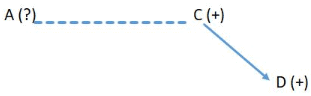Now we should look info. that tells more about A, D or C. The second sentence tells us more about A. So, we’ll incorporate that into the diagram.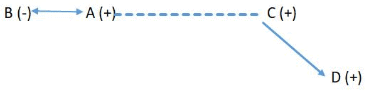Now we shall add the leftover information and the one that fits the most in the current diagram is the relationship between D and E.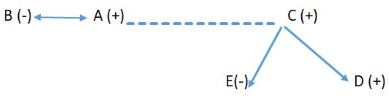We have finally reached in the position of completing our tree diagram by incorporating the final information which is about F who is a female since her husband had died. And B is her daughter-in-law. Therefore, she’s the mother of A and C.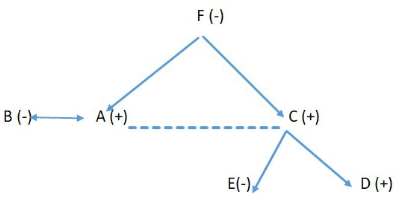Since we are done with the family tree we can easily answer all the above question on the basis of this diagram.

Example: John introduces Mary as the daughter of the only son of my father’s wife. How is Mary related to john?
Solution.
Break the given sentence at as.
Resolving from the last, In the view of john,
My father’s wife – mother
Only son of mother – himself i.e. john
Daughter of john– daughter.

Example. Introducing Suresh, Kalpana said,” His brother’s father is the only son of my grandfather”. How is Kalpana related to Suresh?
Solution.
Break the sentence at is.
From the last up to is: The only son of my grandfather – father
From the first up to is: his brother’s father – father
Suresh’s father = kalpana’s father
So, they are brother & sister.

NOTE
Like in the above type of problems From either ends if it is like Father = Father,  Uncle= Uncle, Mother = Mother, Aunt = Aunt….. Then they both may be brothers/sisters /bother & sister depending upon their sex.

Offer running on EduRev: Apply code STAYHOME200 to get INR 200 off on our premium plan EduRev Infinity!

15 docs|54 tests

,

,

,

,

,

,

,

,

,

,

,

,

,

,

,

,

,

,

,

,

,

;